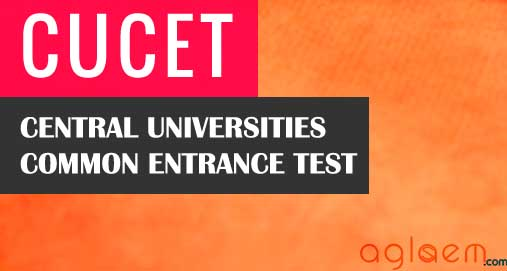### CUCET Sample Question Paper 2015 for All Integrated M.Sc

CUCET Sample Question Paper 2015 is given below.

PART A

1. Rearrange the 5 sentences (numbered P,Q,R,S,T) given below in a meaningful sequence.

P. He did not believe in theoretical ideology.
Q. So he must keep them
R. His principles mainly lay in action.
S. Gandhi Ji had a way of life.
T. He gave much that suits our tradition.

• (a) SPRTQ
• (b) SPQTR
• (c) SPTRQ
• (d) SQPTR

2. Rearrange the 4 sentences (numbered P,Q,R,S) given below in a meaningful sequence.

Sentences 1 and 6 are fixed.

1. Savita was lonely in the house.

P. She was very good at that.
Q. She sat all day in a little room off the main drawing room.
R. She would sit on the rug and do needle work.
S. It was a little room with nothing in it but a few chairs and rug.

6. It was the only thing she had learnt from the Convent School.

• (a) PQRS
• (b) QSRP
• (c) RSPQ
• (d) SRPQ

3. Arrange the activities (P,Q,R,S,T) in a natural sequence.

P: Put on shoes, Q: Wear socks, R: Stand up, S: Sit and bend down, T:Tie shoes laces

• (a) RSTQP
• (b) SRTQP
• (c) RSTPQ
• (d) SQPTR

4. How many triangles are there in the plane figure below?

• (a) 7
• (b) 8
• (c) 9
• (d)10

5. She is a ____________________ girl always _____________________ for knowledge.

• (a) Smart, running
• (b) Tall, running
• (c) School, acquiring
• (d) Studious, eager

PART B

Mathematics

1. In rolling two pair dices the probability of getting sum to be a multiple of 3 is

• (a) 2/3
• (b) ½
• (c) 1/3
• (d) None of these

2. The equation 2

• (a) Circle
• (b) Hyperbola
• (c) Parabola
• (d) Pair of lines

3. Which of the following functions is non-differentiable on R?

• (a) ⃒
• (b) ⃒x ⃒  5/4
• (c) ⃒x ⃒  2/3
• (d) None of them

4. Let A and B be square matrices of order n, then which of the following is incorrect

• (a) C= ABt +BAt is symmetric
• (b) C=AB t – BA t is symmetric
• (c) C=AA t is Symmetric
• (d) det At  – det A

5. The value of α for which the planes x + y + x = 1 and x + 2y + az = 7 are perpendicular to each other

• (a) -9
• (b) 2
• (c) -2
• (d) -3

Physics

1. Two lenses of power -15 D and +15 are in contact with each other.  The focal length of the combination is

• (a) -20 cm
• (b) -10 cm
• (c) +20 cm
• (d) +10 cm

2. In gamma ray emission from a nucleus

• (a) Both the neutron number and the proton number change
• (b) There is no change in the proton number and the neutron number
• (c) Only the neutron number changes
• (d) Only the proton number changes

3. A charged particle moves through a magnetic field perpendicular to its direction. Then

• (a) The momentum changes but the kinetic energy is constant
• (b) Both momentum and kinetic energy of the particle are not constant
• (c) Both momentum and kinetic energy of the particle are constant
• (d) Kinetic energy changes but the momentum is constant

4. In a region, steady and uniform electric and magnetic fields are parallel to  each other. A charged particle is released from rest in this region. The path of the particle will be a

• (a) Circle
• (b) Helix
• (c) Straight line
• (d) Ellipse

5. Which of the following units denotes the dimensions ML2 / Q2 , where Q denotes the electric charges?

• (a) Weber (Wb)
• (b) Wb/m
• (c) Henry (H)
• (d) H/m2

Chemistry

1. For reaction A → B, 80 % A is decomposed in 1 hr. 96% is decomposed in 2 hrs. 99.2% is decomposed in 3 hrs. and 99.84% is decomposed in 4 hrs.  What is the order of reaction?

• (a) First
• (b) Second
• (c) Zero
• (d) Cannot be predicted

2. For an element of FCC crystal lattice having edge length of 400 pm, what will be the maximum diameter of an atom which can be placed in interstitial site so that the structure remains same?

• (a) 117 pm
• (b) 111 pm
• (c) 123 pm
• (d) 129 pm

3. A catalyst is a substance which

• (a) Increases the equilibrium concentration of the product
• (b) Shortens the time to reach equilibrium reaction
• (c) Supplies energy to the reaction
• (d) Increases the equilibrium constant of the reaction.

4. Helium atom is twice heavier than a hydrogen molecule.  At 25o C (298 K), the average kinetic energy of a helium atom is

• (a) Twice that of a hydrogen molecule
• (b) Same as that of hydrogen molecule
• (c) Four times that of a hydrogen molecule
• (d) Half that of a hydrogen molecule

5. The molecule having one unpaired electron is

• (a) NO
• (b) CN
• (c) O2
• (d) CO

Life Science

1. Mannitol is a stored food formed in

• (a) Chlorophyceae
• (b) Phaeophyceae
• (c) Rhodophyceae
• (d) Cyanophyceae

2. Store house of collected plant specimens that are dried, pressed and preserved is called

• (a) Museum
• (b) Herbarium
• (c) Botanical Garden
• (d) Plant biomass

3. Find out the odd among the following

• (a) Cocci
• (b) Vibrio
• (c) Spirulina
• (d) Bacilli

4. Coelom is a body cavity lined by

• (a) Mesoglea
• (b) Ectoderm
• (c) Mesoderm
• (d) Endoderm

5. The major components of cotton fiber is

• (a) Starch
• (b) Protein
• (c) Chitin
• (d) Cellulose